# Dinning room

How many different combinations can we choose if there are 3 soups, 5 kinds of main dish and 2 desserts in the dining room?

Correct result:

n =  30

#### Solution: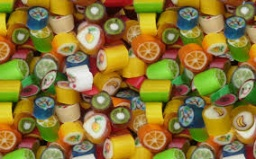We would be pleased if you find an error in the word problem, spelling mistakes, or inaccuracies and send it to us. Thank you!Tips to related online calculators
Would you like to compute count of combinations?

## Next similar math problems:

• Combinations 7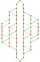A multi-course meal has 3 choices. 3 appetizers, 4 main courses, and 3 desserts. How many different combinations to choose from?
• Glass with icecream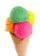We have 6 kinds of ice cream and 5 kinds of fruit. We put 3 cups of ice cream and 2 fruits into each glass. How many can unique decorated glasses be?
• How many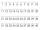How many double-digit numbers greater than 30 we can create from digits 0, 1, 2, 3, 4, 5? Numbers cannot be repeated in a two-digit number.
• DivideHow many different ways can three people divide 7 pears and 5 apples?
• Twenty-fiveHow many three-digit natural numbers are divisible by 25?
• Three-digit numbersWe have digits 0,1,4,7 that cannot be repeated. How many three-digit numbers can we write from them? You can help by listing all the numbers.
• Chocolates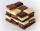In the market we have 3 kinds of chocolates. How many ways can we buy 14 chocolates?
• Telephone numbers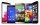How many 7-digit telephone numbers can we put together so that each number consists of different digits?
• Honored students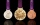Of the 25 students in the class, 10 are honored. How many ways can we choose 5 students from them, if there are to be exactly two honors between them?
• How many 4How many 4 digit numbers that are divisible by 10 can be formed from the numbers 3, 5, 7, 8, 9, 0 such that no number repeats?On the menu are 12 kinds of meal. How many ways can we choose four different meals into the daily menu?From the numbers 1, 2, 3, 4, 5 create three-digit numbers that digits not repeat and number is divisible by 2. How many numbers are there?How many different combinations of two-digit number divisible by 4 arises from the digits 3, 5 and 7?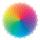Dana confuses sweater and wool has a choice of seven colors. In how many ways she can choose from three colors to the sleeves?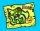There are 4 roads from city A to city B. There are 5 roads from city B to city C. How many different routes can we come from city A to city C via city B?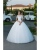We have packed three T-shirts - white, red, orange and five pants - blue, green, black, pink and yellow. How many days can we spend with the old mother if we put on a different combination of clothes every day?There are pages numbered from 2 to 104 in the book. How many digits have to be printed to number the pages?